## Wednesday, August 31, 2016

### On Detecting Signal Spikes with 1D HWT Wavelets: Part 2

Problem

In my previous post, I jotted down a few thoughts on how 1D HWT can be used to detect spikes in signals. Spikes can be broken into two broad categories: up-down spikes and down-up spikes. An up-down spike consists of three structural segments: an upward segment (signal samples increasing), a flat segment (signal samples remain more or less on the same level, i..e, neither increase or decrease), and a downward segment (signal samples decreasing). Similarly, a down-up spike also consists of three structural segments: a downward segment (signal samples decreasing), a flat segment (signal samples neither increasing nor decreasing), and an upward segment (signal samples increasing). In up-down and down-up spikes the flat segments are optional. A specific segment can be detected by analyzing values of 1D HWT wavelets similar to those given in Fig. 8. After a spike is detected, the exact coordinates of the segments can be used to locate a spike to the original signal, e.g., a 2D grayscale image. Detected spikes can subsequently be used to identify various objects in the original signal, i.e., road lanes. In this post, I will outline a few details on how spike detection can be implemented in JAVA.

Spike Class

Below is a JAVA class that implements a spike. It breaks down a spike into three segments (runs) and keeps track of where each run starts and ends both in the wavelet sequences as well as the original signal. I have omitted the getters and setters as well as a few constructors.

public final class OneDHWTSpike {

private double mUpWaveCoeffThresh;            // threshold used to detect upward segments
private double mDownWaveCoeffThresh;       // threshold used to detect downward segments
private int    mNumIters;                                  // number of iterations/scales of 1D HWT
private int    mWaveLengthOfUpRun;              // length of upward run
private int    mWaveLengthOfDownRun;         // length of downward run
private int    mWaveStartOfUpRun;                 // where the upward run starts in the wavelet array
private int    mWaveStartOfDownRun;            // where the downward run starts in the wavelet array
private int    mWaveStartOfFlatRun;               // where the flat run starts in the wavelet array
private int    mWaveLengthOfFlatRun;            // length of the flat run
private int    mSigRowOrCol;                           // the original signal's row/col
private int    mSigRowOrColStart;                  //  the original signal's row/col start
private int    mSigRowOrColEnd;                     // the original signal's row/col end
private int    mSigStartOfUpRun;                    // where the upward run starts in the original signal
private int    mSigLengthOfUpRun;                 // the length of the upward run in the original signal
private int    mSigStartOfDownRun;               // where the downward run starts in the original signal
private int    mSigLengthOfDownRun;            // length of the downward run in the original signal
private int    mSigStartOfFlatRun;                  // where the flat run starts in the original signal
private int    mSigLengthOfFlatRun;               // length of the flat run in the original signal

// rest of code
}

Spike Detection

Below is my JAVA implementation of two methods: the first one implements a possible way to detect up-down spikes; the second one implements detection of down-up spikes. The methods detect spikes in sub-signals taken from rows in images. Column detection is identical.

The first two arguments are the original sub-signal and its 1D ordered HWT. The third argument specifies the number of iterations of 1D ordered HWT. The fourth argument specifies the row of the image from which the sub-signal is taken, the fifth and sixth arguments (sig_col_start and sig_col_end) specify the start and end columns of the sub-signal in the specified row. The arguments 7 - 12 implement the thresholds used in detecting spikes.

The arguments uwc_thresh and dwc_thresh are the thresholds for the HWT wavelets. HWT wavelets below these thresholds do not start up or down runs that constitute spikes. The arguments up_run_len_thresh, down_run_thresh threshold the lengths of the up and down runs, respectively. The arguments up_deg_angle_thresh and down_deg_angle_thresh threshold the angles of the up and down spikes, respectively. The up-down spikes are detected by detecting an up run, then a possible flat run, and then a down run. The down-up spikes are detected by detecting a down run, a possible flat run, and then an up run.

public static ArrayList<OneDHWTSpike>
detectUpDownSpikesInHWTOfRowSigSegmentByWaveDeg(
double[] row_sig_segment, double[] hwt,
int num_iters,
int sig_row, int sig_col_start, int sig_col_end,
double uwc_thresh, double dwc_thresh,
int up_run_len_thresh, int down_run_len_thresh,
double up_deg_angle_thresh, double down_deg_angle_thresh)

{
ArrayList<OneDHWTSpike> spikes = new ArrayList<>();

final int hwt_size = (hwt.length / (int) (Math.pow(2, num_iters)));
final int n = 2 * hwt_size;
int i = hwt_size;

int up_run_start   = -1;
int down_run_start = -1;
int flat_run_start = -1;
while ( i < n ) {

int j = i;
up_run_start = j;
while ( j < n ) {
if ( hwt[j] >= 0 ) { break; }
if (Math.abs(hwt[j]) < uwc_thresh) { break; }
j++;
}

int up_run_len = j - up_run_start;
if (up_run_len < up_run_len_thresh) {
up_run_start = -1; down_run_start = -1; i++;
continue;
}

// detect a flat run
flat_run_start = j;
while ( j < n ) {
if (Math.abs(hwt[j]) >= uwc_thresh || Math.abs(hwt[j]) >= dwc_thresh) { break; }
j++;
}

int flat_run_len = j - flat_run_start;
if (flat_run_len > 0) {
System.out.println("flat_run_len > 0;");
} else {
flat_run_start = -1;
}

if (j + down_run_len_thresh - 1 >= n) {
up_run_start = -1;
down_run_start = -1;
break; // outer while loop
}

down_run_start = j;
while ( j < n ) {
if (hwt[j] <= 0) { break; }
if (hwt[j] < dwc_thresh) { break; }
j++;
}

int down_run_len = j - down_run_start;

if (down_run_len < down_run_len_thresh) {
up_run_start = -1;
down_run_start = -1;
i++;
continue;
}

OneDHWTSpike spike = null;

if (flat_run_len > 0) {
spike = new OneDHWTSpike(sig_row,
sig_col_start,
sig_col_end,
uwc_thresh, dwc_thresh, num_iters,
up_run_start - hwt_size, up_run_len,
flat_run_start - hwt_size, flat_run_len,
down_run_start - hwt_size, down_run_len);

} else {
spike = new OneDHWTSpike(sig_row,
sig_col_start,
sig_col_end,
uwc_thresh, dwc_thresh, num_iters,
up_run_start - hwt_size, up_run_len,
down_run_start - hwt_size, down_run_len);
}
i = j + 1;
}

return spikes;
}

public static ArrayList<OneDHWTSpike>
detectDownUpSpikesInHWTOfRowSigSegmentByWaveDeg(
double[] row_sig_segment, double[] hwt, int num_iters,
int sig_row, int sig_col_start, int sig_col_end,
double uwc_thresh, double dwc_thresh,
int up_run_len_thresh, int down_run_len_thresh,
double up_deg_angle_thresh, double down_deg_angle_thresh)

{
ArrayList<OneDHWTSpike> spikes = new ArrayList<>();

final int hwt_size = (hwt.length / (int) (Math.pow(2, num_iters)));
final int n = 2 * hwt_size;
int i = hwt_size;

int up_run_start   = -1; int down_run_start = -1; int flat_run_start = -1;
while (i < n) {
int j = i; down_run_start = j;
while ( j < n ) {
if (hwt[j] >= 0) { break; }
if (Math.abs(hwt[j]) < dwc_thresh) { break; }
j++;
}

int down_run_len = j - down_run_start;
if (down_run_len < down_run_len_thresh) {
down_run_start = -1;
up_run_start = -1;
i++;
continue;
}

// detect a flat run
flat_run_start = j;
while ( j < n ) {
if (Math.abs(hwt[j]) >= uwc_thresh || Math.abs(hwt[j]) >= dwc_thresh) { break; }
j++;
}
int flat_run_len = j - flat_run_start;
if (flat_run_len > 0) {
System.out.println("flat_run_len > 0;");
} else {
flat_run_start = -1;
}

if (j + up_run_len_thresh - 1 >= n) {
up_run_start = -1;
down_run_start = -1;
System.out.println("j + up_run_len_thresh >= n");
System.out.println("no more spikes");
break; // outer while loop
}

up_run_start = j;
while (j < n) {
if (hwt[j] <= 0) { break; }
if (hwt[j] < uwc_thresh) { break; }
j++;
}

int up_run_len = j - up_run_start;

if (up_run_len < up_run_len_thresh) {
up_run_start = -1;
down_run_start = -1;
i++;
continue;
}

OneDHWTSpike spike = null;

if (flat_run_len > 0) {
spike = new OneDHWTSpike(sig_row, sig_col_start, sig_col_end,
uwc_thresh, dwc_thresh, num_iters,
up_run_start - hwt_size, up_run_len,
flat_run_start - hwt_size, flat_run_len,
down_run_start - hwt_size, down_run_len);

} else {
spike = new OneDHWTSpike(sig_row, sig_col_start, sig_col_end,
uwc_thresh, dwc_thresh, num_iters,
up_run_start - hwt_size, up_run_len,
down_run_start - hwt_size, down_run_len);
}

// a spike filter can be added to detect the strength of spikes
i = j + 1;
}
return spikes;
}

### On Detecting Signal Spikes with 1D HWT Wavelets: Part 1

Problem

Suppose that we have a 1D signal where we need to detect spikes, i.e., significant changes in signal values. Of course, what makes a given change significant is domain- or problem-dependent. 1D HWT can be used to detect spikes. In this post, I will write down a few thoughts on how one can define spikes conceptually. In the next post, I will detail a JAVA implementation of detecting spikes with 1D HWT.

Sample Images & Signals

Let us take a look at a concrete image where spikes enable us to detect useful features. Fig. 1 gives an image of a road with two lanes. The original image has been grayscaled and blurred with Gaussian blur using a 7x7 window for denoising. We can take a 1D horizontal sub-signal from this image (or several such sub-signals) and use 1D HWT of that subsignal to detect spikes.Figure 1. Sample road image
Fig. 2 gives a graphical representation of a subsignal. Specifically, the horizontal white line is a row from which a signal of 128 samples is taken at row 87 on the left of the image, i.e., from columns 0 to 127. The two vertical lines represent the beginning and the end of a spike detected over the left lane. Fig. 3 depicts another signal of 128 samples taken at the same row on the right side of the image and a spike detected over the right lane.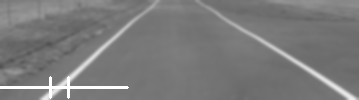Figure 2. Signal of 128 samples from row 87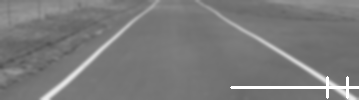Figure 3. Signal of 128 samples from row 87

1D HWT Analysis of Signals for Spike Detection

Fig. 4 is an graph of grayscale values in the signal depicted as the horizontal line in Fig. 2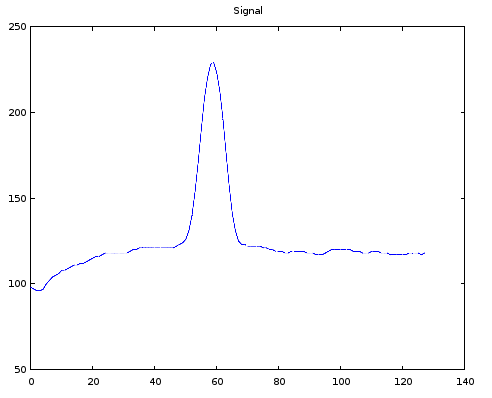Figure 4. Graph of grayscale values of 128 sample signals in Fig. 2

If we apply 1 scale of 1D Ordered HWT to this signal, we get the graph shown in Fig. 5. The first 64 values represent a downsampled signal whereas the remaining 64 values are the corresponding Haar wavelet coefficients.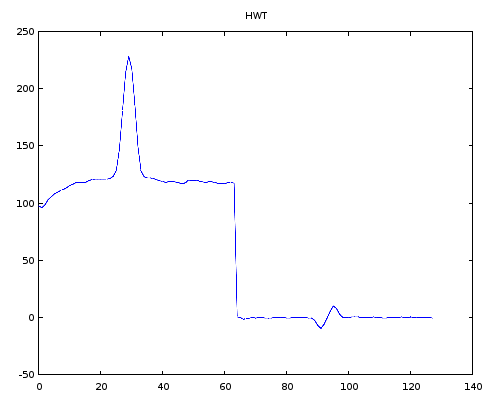Figure 5. 1 scale of 1D HWT of the signal in Fig. 4

Figures 6 and 7 gives graphs of the two parts of Fig. 5, i.e., the downsampled signal and the corresponding wavelets.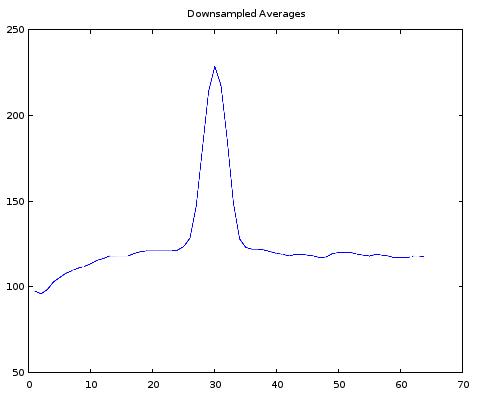Figure 6. Downsampled signal after 1 scale of 1D HWT of the signal in Fig. 4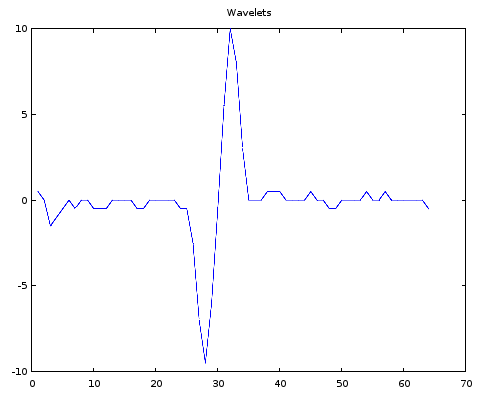Figure 8. Haar wavelets after 1 scale of 1D HWT of the signal in Fig. 4

Definition of Spikes

1D HWT wavelets can be used to detect spikes. Spikes can be broken into two broad categories: up-down spikes and down-up spikes. An up-down spike consists of three structural segments: an upward segment (signal samples increasing), a flat segment (signal samples remain more or less on the same level, i..e, neither increase or decrease), and a downward segment (signal samples decreasing). Similarly, a down-up spike also consists of three structural segments: a downward segment (signal samples decreasing), a flat segment (signal samples neither increasing nor decreasing), and an upward segment (signal samples increasing). In up-down and down-up spikes the flat segments are optional. A specific segment can be detected by analyzing values of 1D HWT wavelets similar to those given in Fig. 8. After a spike is detected, the exact coordinates of the segments can be used to locate a spike to the original signal, e.g., a 2D grayscale image. Detected spikes can subsequently be used to identify various objects in the original signal, i.e., road lanes.